# What is Optical Amplifier Noise Figure?

All amplifiers degrade the signal-to-noise ratio (SNR) of the amplified signal because of spontaneous emission that adds to the signal during its amplification.

The SNR degradation is quantified through a parameter Fn, called the amplifier-noise figure in analogy with the electronic amplifiers, and is defined as

(Equation 1.12)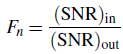where SNR refers to the electrical power generated when the signal is converted to electrical current by using a photodetector.

In general, Fn would depend on several detector parameters which govern the shot noise and thermal noise associated with the detector. One can obtain a simple expression for Fn by considering an ideal detector whose performance is limited by shot noise only.

Consider an amplifier with the amplification factor G so that the output power is related to the input power by Pout = GPin. The SNR of the input signal is given by

(Equation 1.13)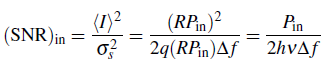where <I> = RPin is the average photocurrent, R=q/hν is the responsivity of an ideal photodetector with unit quantum efficiency, and

(Equation 1.14)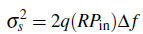is obtained for the shot noise by setting the dark current Id = 0. Here Δf is the detector bandwidth. To evaluate the SNR of the amplified signal, one should add the contribution of spontaneous emission to the receiver noise.

The spectral density of spontaneous-emission-induced noise is nearly constant (white noise) and can be written as

(Equation 1.15)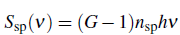where ν is the optical frequency. The parameter nsp is called the spontaneous-emission factor or population-inversion factor. Its value is 1 for amplifiers with complete population inversion (all atoms in the excited case), but becomes >1 when the population inversion is incomplete.

For a two-level system

(Equation 1.16)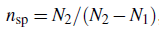where N1 and N2 are the atomic populations for the ground and excited states, respectively.

The effect of spontaneous emission is to add fluctuations to the amplified power which are converted to current fluctuations during the photodetection process.

It turns out that the dominant contribution to the receiver noise comes from beating of spontaneous emission with the signal itself. This beating phenomenon is similar to heterodyne detection: Spontaneously emitted radiation mixes coherently with the amplified signal at the photodetector and produces a heterodyne component of the photocurrent. The variance of the photocurrent can then be written as

(Equation 1.17)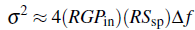The SNR of the amplified signal is then given by

(Equation 1.18)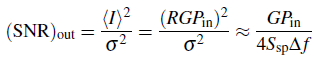where the last relation was obtained by neglecting the term due to shot noise and is valid for G >> 1.

The amplifier-noise figure can now be obtained by substituting Eqs. 1.13 and 1.18 in 1.12. It we also use Eq. 1.15 for Ssp, we obtain

(Equation 1.19)This equation shows that the SNR of the amplified signal is degraded by a factor of 2 (or 3dB) even for an ideal amplifier for which nsp = 1. For most practical amplifiers, Fn exceeds 3 dB and can be as large as 6-8 dB. For its application in optical communication systems, an optical amplifier should have as low an Fn as possible.

The effective noise figure of the chain of cascaded optical amplifiers can be calculated as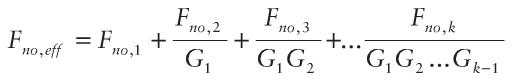where Fno,eff is the effective noise figure of the amplifier chain that contains the total number of k optical amplifiers. The first amplifier in the chain is the most important one in terms of the noise impact. That is the reason why multistage optical amplifiers should be designed to have the first stage with lower noise figure.

Accordingly, any decrease in the effective value of the amplifier’s noise figure will bring a significant benefit in the overall system performance.

The total power of the spontaneous emission noise is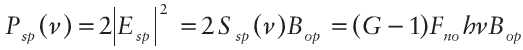where Esp is the electric field of the spontaneous emission, Bop is the effective bandwidth of spontaneous emission determined either by the optical amplifier bandwidth or by an optical filter. Please note that factor 2 in the equation accounts for the contributions of two fundamental polarization modes, which are present at the output of the optical amplifier.